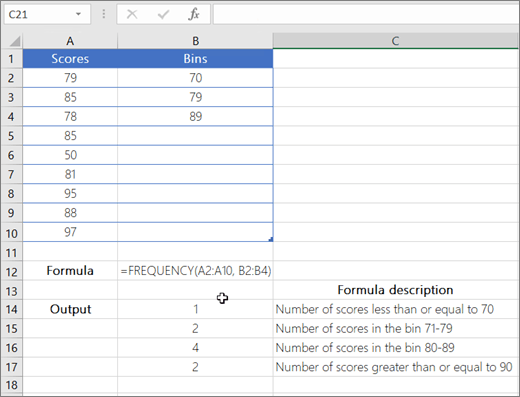# Why you need to use the FREQUENCY Function?

• Applies to: Excel for Microsoft 365,Excel for Microsoft 365 for Mac, Excel for the web, Excel 2019, Excel 2016, Excel 2019 for Mac, Excel 2013, Excel 2010, Excel 2007, Excel 2016 for Mac, Excel for Mac 2011, Excel Starter 2010.

This post will inform you why it is useful to use the FREQUENCY function in Microsoft Excel. It will cover the meaning of this function, its formula syntax, vital conditions when using it, and also an example to see how it actually works in practice.

Put simply, the FREQUENCY function calculates how often values recur among a range of values, and then captures a vertical array of numbers. For example, use FREQUENCY to count the total number of test scores that lie within ranges of scores. Because FREQUENCY yields an array, you must type it as an array formula.

## Syntax of the FREQUENCY Function

FREQUENCY(data_array, bins_array)

The FREQUENCY function syntax has the following arguments:

• data_array   – Required. An array of or reference to a range of values for which you seek to count frequencies. If data_array has no values, FREQUENCY yields an array of zeroes.
• bins_array  –  Required. An array of or reference to intervals into which you intend to organise the values in data_array. If bins_array includes no values, FREQUENCY captures the number of elements in data_array.

## Remarks of the FREQUENCY Function

Note: If you have a current version of Microsoft 365, then you can simply enter the formula in the top-left-cell of the output range, then press ENTER to confirm the formula as a dynamic array formula. Otherwise, the formula must be entered as a legacy array formula by first choosing the output range. Next, you input the formula in the top-left-cell of the output range. After this, you then press CTRL+SHIFT+ENTER to verify it. Excel automatically adds curly brackets at the start and end of the formula without you manually having to insert them. Also, for more information on array formulas, see Guidelines and examples of array formulas.

• The number of elements in the captured array is one more than the number of elements in bins_array. The additional element in the gathered array returns the count of any values greater than the highest interval. For example, when counting three ranges of values (intervals) that are put into three cells, make sure you enter FREQUENCY into four cells for the outcomes. The surplus cell brings back the number of values in data_array that exceed the third interval value.
• Furthermore, FREQUENCY omits empty cells and text.

## Example## Need more help?

You can always ask an expert in the Excel Tech Community, get support in the Answers community, or suggest a new feature or improvement on Excel User Voice.

## Similar Statistical Function Topics

Find the middle value with MEDIAN

Rank data without sorting with RANK

Return the largest value in a data set with LARGE

Return the smallest value in a data set with SMALL

Tabulate blank cells with COUNTBLANK

Build analysis via regression techniques with TREND

GROWTH function

All statistical functions